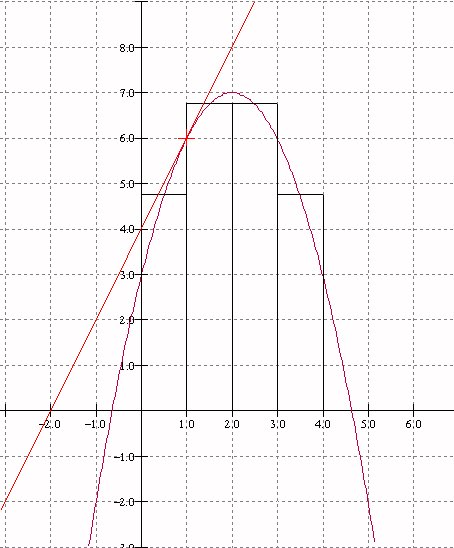Tuesday,  April  25, 2006
Algebra
for finding Rates and Slopes: the "derivative"
for finding areas and position change: the "integral"
The Fundamental Theorem of Calculus

Recall from last class:

Growth Rates: m - linear
Graphing and rates: slopes of lines, approximating curves with lines.
slope of straight line:
m =  (change in y)/(change in x)
Problem: Find the Area "under a straight line":
Motion: time: t ; position:s
constant and average velocity:
v = (change in s)/(change in t)
Problem: Given a constant velocity or a constant acceleration for a moving object, find the distance travelled.
Note: The motion of a falling object is perhaps the simplest physical example of motion with a variable velocity. Such an object has a constant acceleration.
Questions:
What is the "slope" on a curved line?
What is the instaneous velocity of a falling object?
What is the area of a region in the plane bounded by a curved line?
How far will
a moving object travel in a fixed interval of  time?

Review Activity: Estimations of slopes of tangent lines and area for a parabola given by a quadratic relation between coordinates y and x."zooming"
Example: What line does the graph of  y = x2 look like near (3,9).

Related measurement concepts:
• Instantaneous Rate of growth or motion.
• Slope (gradient) of a curved line, tangent line to a curve.
• Net Change in measured variable based on rate of growth.
• Area of a region in the plane.

What is the calculus?
A systematic method for answering these questions of velocity, tangents, change in position and area that uses the algebraic representation of a relation between y and x .
Examples: if y = .... then the slope of the tangent to the curve described by this relationship at the point (a,b) is....
If the position s = ... t.... then the instantaneous velocity of the object moving to correspond to this relation  at time t is .................
If the velocity of a moving object is given by v= .... t..... , then the object has a net change during the interval from t = a to t = b of .....
the area of the region in the plane bounded by the Xis, the lines X = a and X= b and the graph of y= ...x... is....

Newton: Tangent lines, velocity, and the derivative.
Using systematic  estimates to find i) the slope of the tangent line and ii) the instantaneous velocity.
The derivative: a limiting number interpreted as
i) the slope of the tangent line or
ii) the instantaneous velocity
A calculus for derivatives: core functions- example powers of x.
Rules: sum . constant multiples, etc. [Ways that we put together core functions]
Activity: Finding the derivative of a function by estimation.
Application: Finding maximum - old Macdonald: Fence Problem- find dimension of largest rectangular area enclosed by a fence of length 100 meters using the side of a barn as part of the boundary of the rectangle.
A = f (x).
What does the maximium area have to do with the slope of the tangents to the graph of A?
When is tangent horizontal? calculus - formula for slope of the tangent as a function of x: ...

Notation: y = f(x). dy/dx = f'(x) Leibniz.Lagrange

Reversing  the process- given the derivative- find the original function(s): "Integration".
Interpretation: Given slopes of tangent lines- fit a curve to them. [A dot to dot problem.]
Given velocity, find a position motion that corresponds. [A detective problem]
Determining position and areas. Change in position - area of rectangle.
Putting concepts together with computations.
To find area under curve y = v(x). Find s where s' = v. Area corresponds to net change in position.
Fundamental Theorem of Calculus: Area = s(b) - s(a).

Notation: v = F '(x); int v dx = F(x) + C; int_a^b v dx = F(b) - F(a)